# Perfect Squares and Square Roots Lesson Plan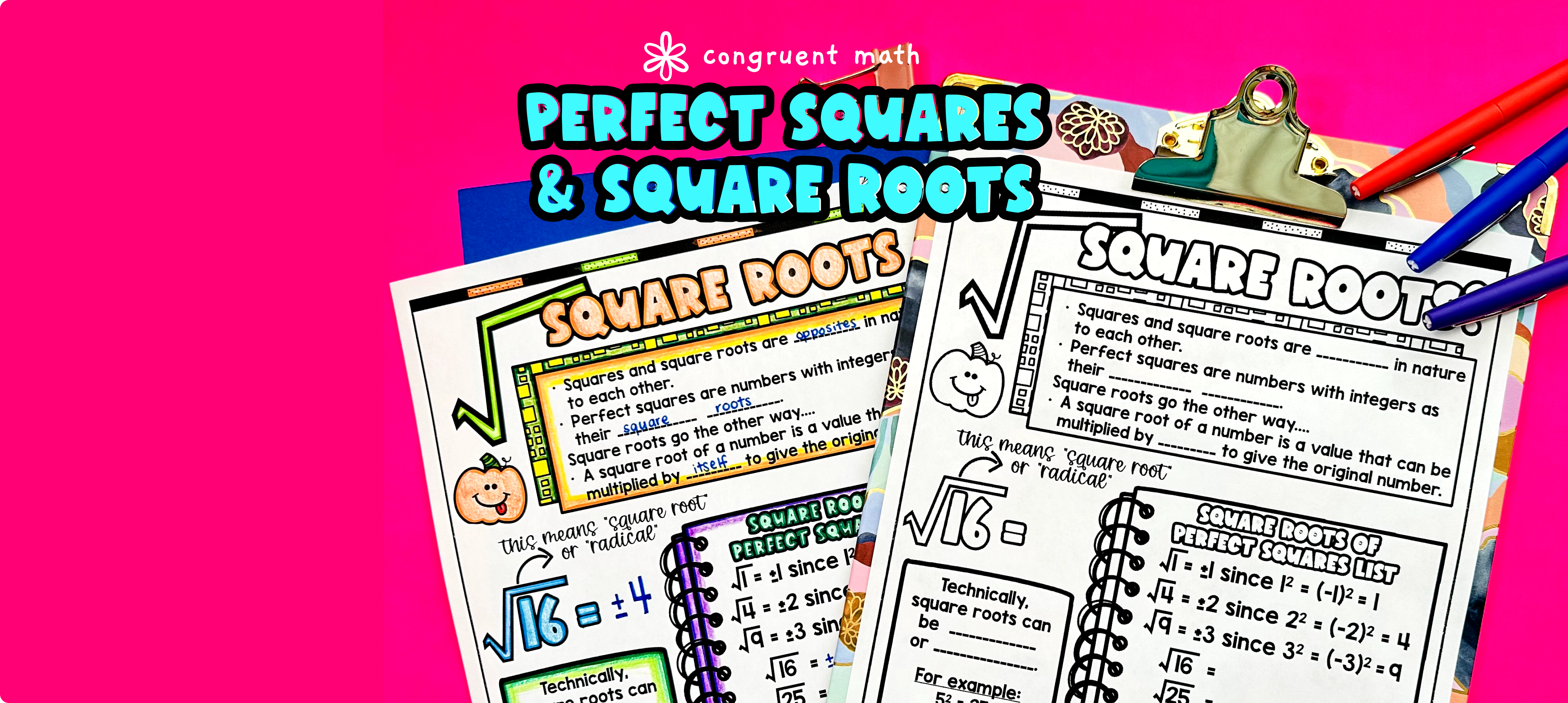Pin This

## Overview

Ever wondered how to teach perfect squares and square roots in an engaging way to your eighth grade students?

In this lesson plan, students will learn about perfect squares and square roots and their real-life applications. Through artistic, interactive guided notes and practice worksheets including a color by code activity, students will gain a comprehensive understanding of perfect squares and square roots.

The lesson culminates with a real life example that explores how designers use perfect squares and square roots to design bathroom tiles.

## Get the Lesson Materials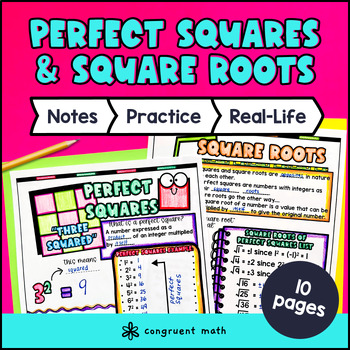\$4.25

## Learning Objectives

After this lesson, students will be able to:

• Define perfect squares and square roots

• Identify perfect squares and square roots of given numbers

• Apply the concept of perfect squares to solve equations in the form x² = p

• Explain a real-life math application involving perfect squares and square roots

## Prerequisites

Before this lesson, students should be familiar with:

• Basic multiplication and division skills

• Understanding of the concept of square numbers

• Basic understanding of exponents and how they relate to repeated multiplication

• Understanding of the concept of radicals and square roots

• Ability to perform basic operations with rational numbers (optional, but helpful)

## Key Vocabulary

• Perfect squares

• Square roots

• Equations

• Rational numbers

## Procedure

### Introduction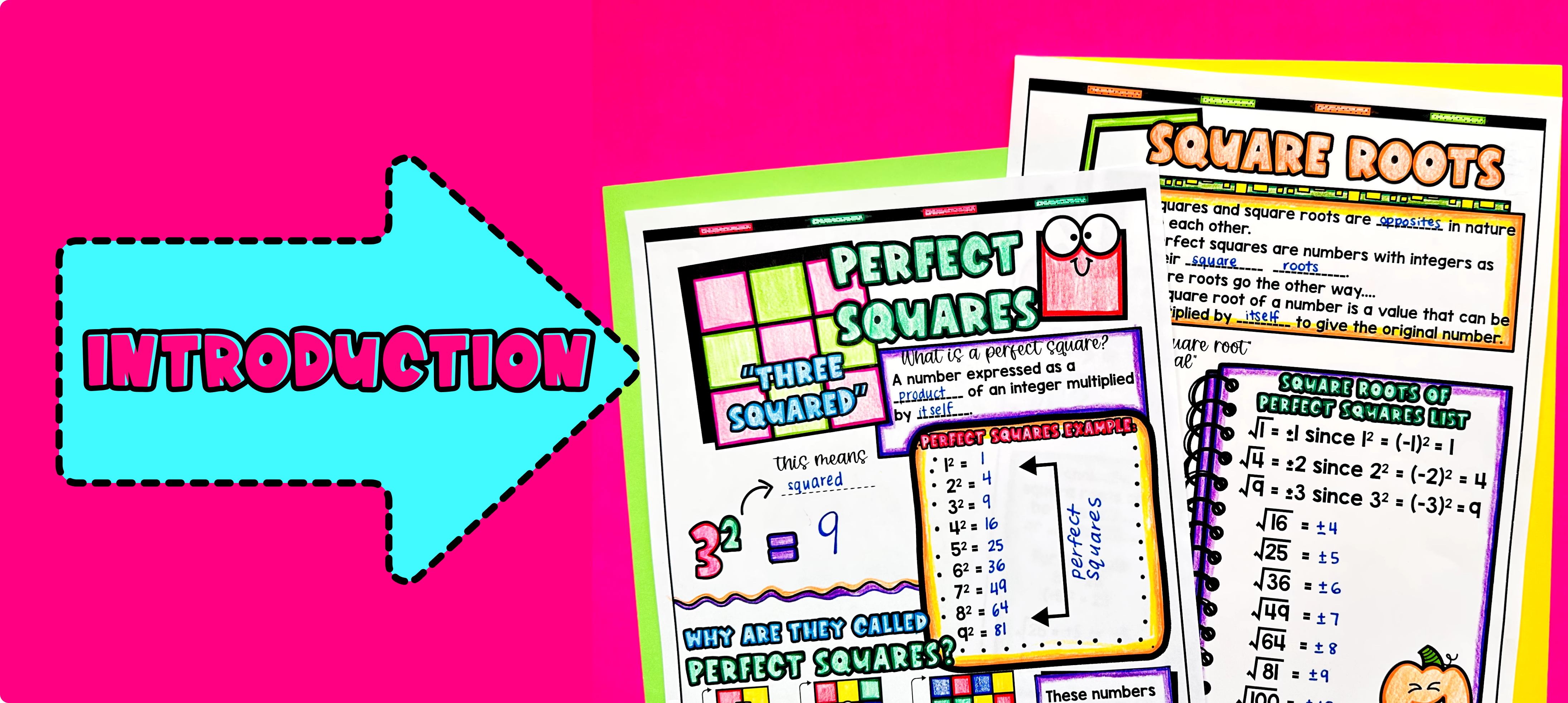Pin This

As a hook, ask students why designers need to understand perfect squares and square roots when designing bathroom tiles. Refer to the real-life application on the last page of the guided notes for ideas.

Use the first page of the guided notes to introduce perfect squares. Walk through the key points of identifying perfect squares and the properties of perfect squares. Refer to the FAQs for a walk-through on this, as well as ideas on how to respond to common student questions.

Continue using the guided notes to introduce square roots. Walk through the key points of square roots and how to find the square root of a perfect square. Refer to the FAQs for a walk-through on this, as well as ideas on how to respond to common student questions.

Based on student responses, reteach concepts that students need extra help with. If your class has a wide range of proficiency levels, you can pull out students for reteaching, and have more advanced students begin work on the practice exercises.

### Practice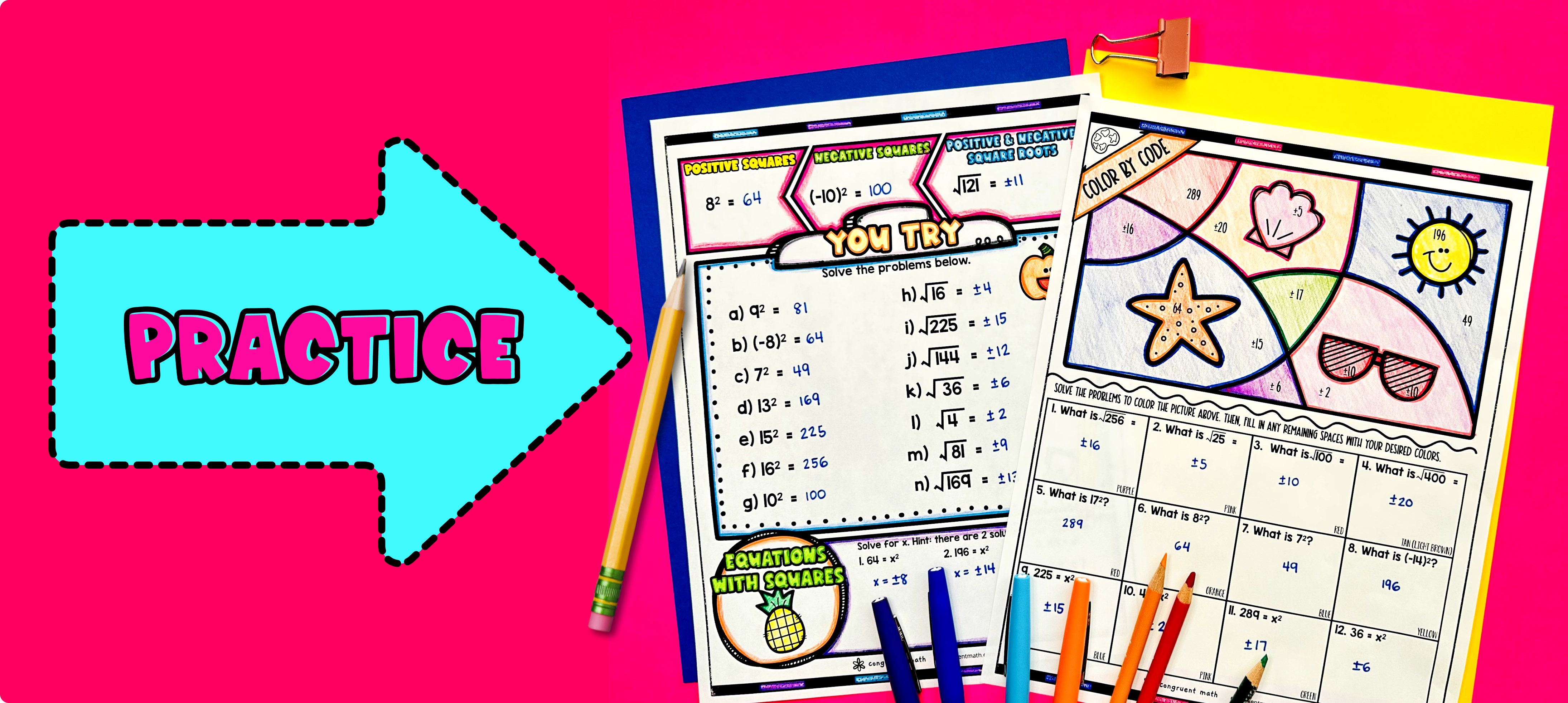Pin This

Have students work through the problems on the "You Try" page, either collaboratively or independently. Walk around and spot-check student answers on the check for understanding activity.

Fast finishers can dive into the color by code activity included in the resource for extra practice. You can assign it as homework for the remainder of the class.

### Real-Life Application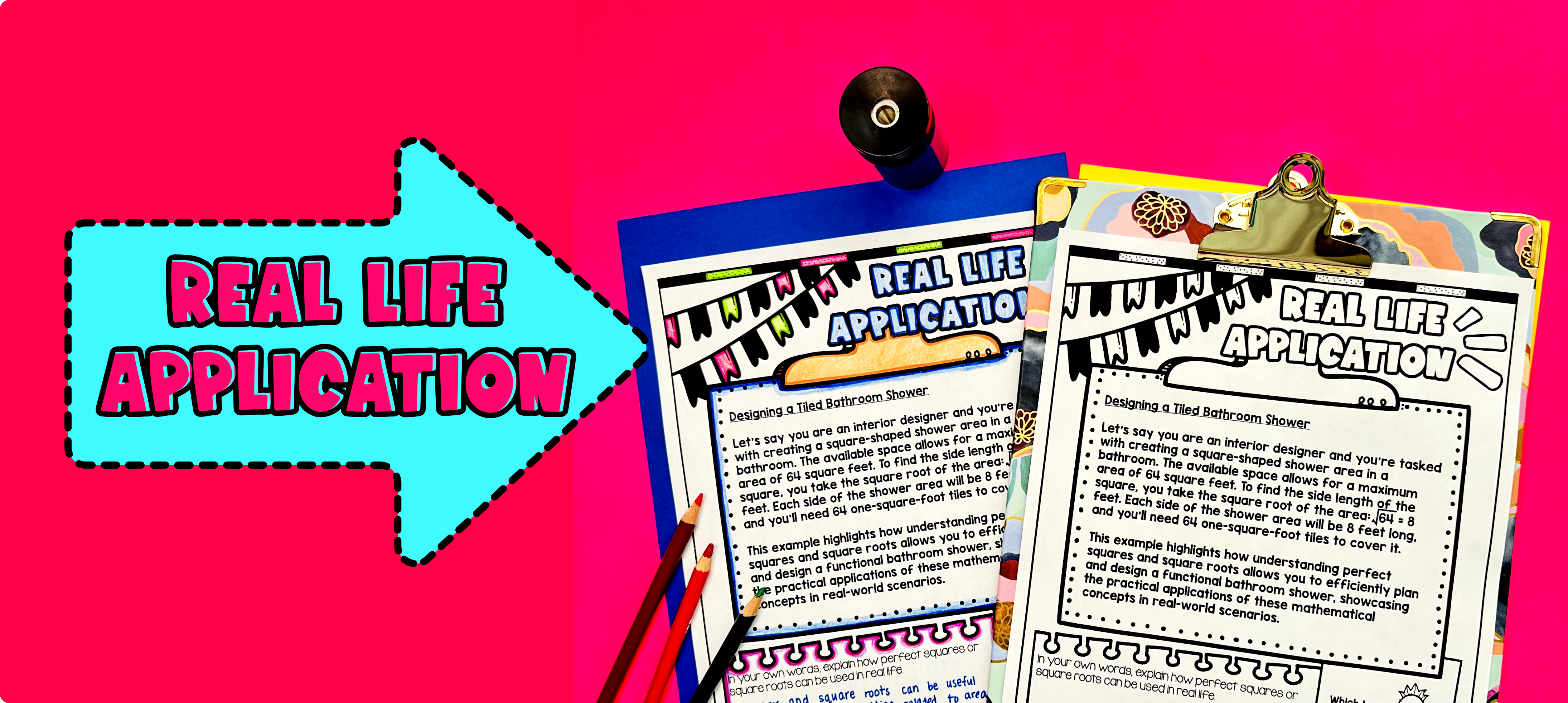Pin This

Bring the class back together, and introduce the concept of applying perfect squares and square roots in real-life scenarios. Explain to the students that understanding perfect squares and square roots can be useful in various situations, such as designing bathroom tiles.

To engage the students, ask them to imagine that they have been hired as interior designers to create a pattern for a set of bathroom tiles. Explain that they need to come up with a design that uses perfect squares and square roots to determine the dimensions of the tiles.

Encourage students to think about how they can use the concept of perfect squares and square roots to ensure that the tiles fit well and create an aesthetically pleasing pattern. Emphasize the importance of accurately calculating the dimensions of the tiles using square roots.

Refer to the FAQ or additional resources for more ideas on how to teach the real-life application of perfect squares and square roots.

## Extensions

If you’re looking for digital practice for perfect squares, square roots, and related concepts, try the Pixel Art activities in Google Sheets. Every answer is automatically checked, and correct answers unlock parts of a mystery picture. It’s incredibly fun, and a powerful tool for differentiation.

Here is an activity to explore: